×

×

ASU / Physics / PHY 112 / What is the purpose of maxwell’s equations?

# What is the purpose of maxwell’s equations? Description

##### Description: This study guide covers what is going to be on our third midterm.
7 Pages 39 Views 2 Unlocks
Reviews

sanam.arefi (Rating: )

PHY 112 Exam 3 Study Guide (Lectures 13-18)

## What is the purpose of maxwell’s equations?Physics 112 with Professor Covatto

Lecture 13

Key Ideas: Maxwell’s equations, the EM spectrum, speed of EM waves, energy transport, Doppler Effect

1. Maxwell's Equations

a. Gauss’s Law

i. Electric fields (not induced) must begin on positive charges and end on negative charges

b. Gauss’s Law for magnetism

i. There are no magnetic monopoles----a magnet must have at least one north and south pole

i. A changing magnetic field creates an electric field

d. Ampere-Maxwell Law Don't forget about the age old question of Is bayreuth worth visiting?

i. A current or a changing electric field creates a magnetic field

2. EM waves

a. When Maxwell’s equations are combined; magnetic field vary with position and time, and solutions are electric.

## What is the content of maxwell’s equations?b. EM waves in vacuum travel at the speed of light ©

c. electric and magnetic fields oscillate in phase

i. Related through ƛf = c

d. EM waves are transverse; the magnetic fields travel in a direction that is perpendicular to the direction in which the wave will travel If you want to learn more check out What is the meaning of experimental design?

3. Doppler Effect

a. Equation: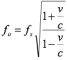Lecture 14

## How to calculate the doppler effect?Key Ideas: Huygens’s principle, reflection, refraction, total internal reflection, polarization 1. Huygens’s Principle

a. Wavefront- a set of points with equal phase

b. At some time t, consider every point on a wavefront as a source of a new spherical wave. These wavelets move outward at the same speed as the original wave. At a later time t+Δt, each wavelet has a radius vΔt, where v is the speed of propagation of the wave. The wavefront at t+Δt is a surface tangent to the wavelets

2. Ray- is perpendicular to the wavefronts, and points in the direction of wave propagation; a line in the direction along which light energy is flowing If you want to learn more check out What is the meaning of violent motion?

3. Geometric Optics

a. Approximation to the light’s behavior that applies when diffraction and interference are negligible

b. For diffraction to be negligible, compared to the wavelength of light, the sizes of the objects must be large

4. Reflection of Light

a. Specular reflection- when light is reflected from a smooth surface, the rays incident, at a given angle, are reflected at the same angle

b. Angle of incidence=angle of reflection

5. Refraction of Light

a. When light rays go from one medium to another, they change direction 6. Snell’s Law: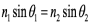We also discuss several other topics like What are unit of account costs?

7. Dispersion

a. When some materials, that have an index of refraction, depend on the wavelength of light

8. Polarization

a. If a string has vibrations on the same plane in which it is linearly polarized, then the orientation of the plane will determine the polarization state of the wave b. A polarizer will transmit linear polarized waves-in the same direction independent of the incoming wave

Lecture 15

Key Ideas: Formation of images, plane mirrors, spherical mirrors

1. Formation of images

a. Real image- if light rays from a point on the object converge to a corresponding point on the image. Ex- camera lens

b. Virtual image- if light rays form a point on the object are directed as if they diverged from a point on the image, even though the rays do not actually pass through the image point

2. Plane mirrors

a. A point source and its image are at the same distance from the mirror, but on opposite sides of the mirror

3. Spherical Mirrors

a. Mirror will curve away from observer

b. Concave mirror- curves toward the observer

c. Expression for magnification: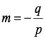We also discuss several other topics like How can some things be the same entity but still be separate?

d. Mirror Equation: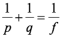Lecture 16

Key Ideas: Apparent Depth, thin lenses, combinations of lenses and mirrors 1. Apparent Depth

a. Snell’s Law: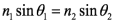b. Actual depth and defect can be related by: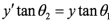If you want to learn more check out How many levels of reality are there according to plato?

2. Thin lenses

a. Diverging lens- will bend light away from principal axis

b. Converging lens- will bend light toward principal axis

c. Equation: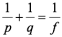3. Combination of Lenses

a. Image formed by one lens is the object for next lens

b. Equation: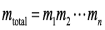Lecture 17

Key Ideas: The camera, the eye, the magnifier, the compound microscope, the telescope 1. The Camera

a. Forms a real, inverted image

b. For objects far away, the film must be placed one focal length away from lens 2. The eye

a. Lens is at a fixed distance from retina

b. Lens has a variable focal length-adjusted to keep image distance constant, as object distance changes

c. Near point- closest distance from eye that an object can be seen clearly d. Far point- farthest distance from eye that an object can be seen clearly

e. Refractive power of a lens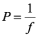3. The simple magnifier

a. If object is farther from eye, it will smaller

b. For object to look bigger, it’s image formed on retina must be made bigger c. Angular size of object: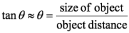4. Compound Microscope

a. Two converging lenses used to produce highly magnified image

b. Objective lens-forms enlarged real image

c. Eyepiece- used to view image

d. Equation: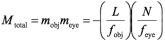5. Telescope

a. Combination of lenses and/or mirrors used to collect a large amount of light and bring it to a focus

b. Refracting telescope uses lens

c. Barrel length: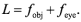d. Angular magnification: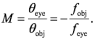Lecture 18

Key Ideas: Constructive and destructive interference, thin films, Young’s double slit experiment 1. Constructive and destructive interference

a. Coherent waves- when waves maintain fixed phase relationship

b. Incoherent waves- if they have any other relationship, and are from different sources

c. Constructive interference- when two waves are in phase

i.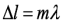d. Destructive interference- when two are a half cycle out of phase

i.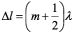2. Thin films

a. The reflected wave is inverted, when incident light ray reflects from a boundary with a higher index of refraction

b. Within a medium, a light ray can be reflected many times

3. Young’s double slit experiment

a. Place a source of coherent light behind a mask that has two vertical slits cut into it. The slits are L tall, their centers are separated by d, and their widths are a. b. Slits become sources of waves that can interfere with each other, as they travel outward

****END OF STUDY GUIDE****

Page ExpiredIt looks like your free minutes have expired! Lucky for you we have all the content you need, just sign up here